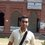MAYBE a familiar problem

I have several problems. One of them is: Find is the rightmost digit of 1+4^1+4^2+…4^2012 As it's my 1st discussion I started by picking only 1 problem...Note by Sheikh Asif Imran Shouborno
6 years, 4 months ago

This discussion board is a place to discuss our Daily Challenges and the math and science related to those challenges. Explanations are more than just a solution — they should explain the steps and thinking strategies that you used to obtain the solution. Comments should further the discussion of math and science.

When posting on Brilliant:

• Use the emojis to react to an explanation, whether you're congratulating a job well done , or just really confused .
• Ask specific questions about the challenge or the steps in somebody's explanation. Well-posed questions can add a lot to the discussion, but posting "I don't understand!" doesn't help anyone.
• Try to contribute something new to the discussion, whether it is an extension, generalization or other idea related to the challenge.
• Stay on topic — we're all here to learn more about math and science, not to hear about your favorite get-rich-quick scheme or current world events.

MarkdownAppears as
*italics* or _italics_ italics
**bold** or __bold__ bold

- bulleted
- list

• bulleted
• list

1. numbered
2. list

1. numbered
2. list
Note: you must add a full line of space before and after lists for them to show up correctly
paragraph 1

paragraph 2

paragraph 1

paragraph 2

> This is a quote
This is a quote
# I indented these lines
# 4 spaces, and now they show
# up as a code block.

print "hello world"
# I indented these lines
# 4 spaces, and now they show
# up as a code block.

print "hello world"
MathAppears as
Remember to wrap math in $$...$$ or $...$ to ensure proper formatting.
2 \times 3 $2 \times 3$
2^{34} $2^{34}$
a_{i-1} $a_{i-1}$
\frac{2}{3} $\frac{2}{3}$
\sqrt{2} $\sqrt{2}$
\sum_{i=1}^3 $\sum_{i=1}^3$
\sin \theta $\sin \theta$
\boxed{123} $\boxed{123}$

Sort by:

1

as 1+4^1+4^2+…4^2012 = 1+4+6+...+6 = 1+0+....0 = 1

- 6 years, 4 months ago

Take a look at this. $4^1=4$ , $4^2=16$, $4^3=64$, $4^4=256$. In other words, $4^{odd}= ...4$ and $4^{even}= ...6$. So, $4^{odd}+4^{even}=...10$ Now we are going to take the terms in pairs. $1+(4^1+4^2)+...+(4^{2011}+4^{2012})$ We know that terms inside the brackets are going to have $0$ as the rightmost digit. And adding all of them up together will have $0$ as the rightmost digit. So if we add the $1$ that is outside the bracket (the leftmost $1$), the last digit's going to be $1$. Hope this helps!

- 6 years, 4 months ago

A slightly cleaner way (which is still saying that same thing) is to say that for $n\geq 0$, $4^{2n+1} + 4^{2n+2} = 4^{2n} ( 4 + 16) = 4^{2n} \times 20$, hence has a units digit of 0.

This avoids the slight issue of $4^0 =1$, while claiming that $4^{even} = \ldots 6$.

Staff - 6 years, 4 months ago

Thank you for pointing it out.

- 6 years, 4 months ago

Consider the following: $4^{2k}\equiv 4{\pmod{10}}$ and $\\4^{2k+1}\equiv 6{\pmod{10}}$  Therefore $1+4^1+4^2+4^3...4^2012 \equiv \pmod{10}$ Observe all the $4's$ and $6's$ pair up and we get the remainder $1$ mod 10. So the rightmost digit is $1$

- 6 years, 4 months ago

Latex error: It should be $1+4^1+4^2+4^3...4^{2012}≡ 1+4+6+4+6.....\pmod{10}$

- 6 years, 4 months ago

right

- 6 years, 4 months ago

Ignoring 1, if we start with 4^1, we will be able to recognise that 4^(odd number) has the last digit as 4, for eg:-4^3 = 64 and 4^5=1024. In the same way, 4^(even number) has the last digit as 6. The sum is congruent to 0(mod10). Now adding 1 in the sequence, we will get the sum congruent to 1(mod10). So 1 is the last digit.

- 6 years, 4 months ago

Another method to solve this problem, is to observe that the numbers are in geometric progression. So use the sum formula of geometric progression and try to find the last digit either by simple calculation, or modular arithmetic.

- 6 years, 4 months ago